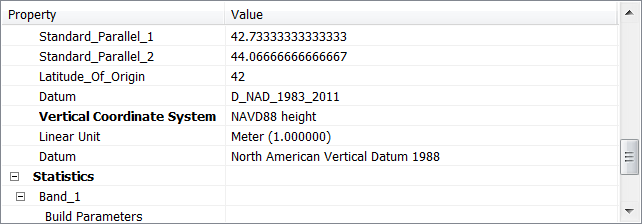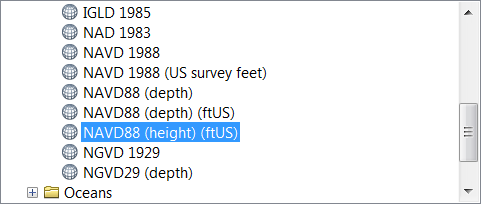# Converting NAVD88 linear units from Meter to Feet

1631
1
09-18-2017 07:24 AM
Labels (1)
Occasional Contributor II

I have a raster DEM that has the vertical coordinate system NAVD88 (height) with linear units Meter.I would like to convert the linear units to US_Feet. As far as I know, ArcGIS does not support conversion between vertical datum. Since the datum remains the same (NAVD88), I assume I can use raster calculator to apply the following transformation to each cell value:

Value * (39.37/12)                  {Convert linear unit from Meter to US_Feet)

However, the metadata would still state that the units are in Meter.

So, I have a few questions about converting linear units of a vertical datum. Is applying the above transformation valid? How does this affect the vertical datum stored in the metadata (i.e. if I were to now use VDATUM to convert to NAVD88 to NGVD29, how would my cell values be affected)? After using raster calculator, can I just re-define the projection (using define projection tool) to from NAVD88 (height) to NAVD88 (height) (ftUS)?Thank you!

Tags (2)
by